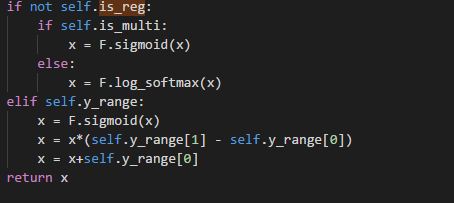# I still don't know how to perform model.fit(X,Y) in Fast.ai

Prior to fast.ai, I’ve mainly acquainted myself with scikit-learn and Keras and have been used to manually cleaning the data into X and Y and calling fit() on a model object.

I’m a little embarrassed to admit I still don’t know how to put together a minimal neural network in Fast.ai and use it to fit a generic dataset, the way you call fit() in sklearn or keras.

Let’s say I have a dead simple dataset like the following, how would I fit it using Fast.ai?

``````import numpy as np
X = np.array([3,5,6,1,32,2,9,22,14,5])
Y = np.array([45,23,44,5,71,2,33,1,12,13])
``````

What’s Fast.ai’s equivalent of a bare-minimum model for fitting X and Y?

I see that in Lesson 3 (Rossman), there were some steps like the following in the “DL” section:

``````md = ColumnarModelData.from_data_frame(PATH, val_idx, df, yl.astype(np.float32), cat_flds=cat_vars, bs=128, test_df=df_test)
cat_sz = [(c, len(joined_samp[c].cat.categories)+1) for c in cat_vars]
emb_szs = [(c, min(50, (c+1)//2)) for _,c in cat_sz]
m = md.get_learner(emb_szs, len(df.columns)-len(cat_vars),
0.04, 1, [1000,500], [0.001,0.01], y_range=y_range)
<...  skip ... >

m.fit(lr, 3, metrics=[exp_rmspe])
``````

What’s the simplest model in the Fast.ai stack that’ll fit X and Y here without any frills (and we can get to the frills later once we figure that the fit is imperfect)?

You have the source already, isn’t it? Just use from_array instead of from_data_frames.
You still have to specific the embedding size.

If you dive into the source code, you will notice that the learner is a class built on top of nn.Module(PyTorch). If you just want a single hidden layer, you should use nn.Module(PyTorch) instead of fast.ai library. As the model in fast.ai is not a simple one (usually), that’s part of the reason why you have to specific some more hyperparameters, as the model consists multiple blocks usually (Which fastai try to build a good default model)

In the case of ColumnarModelData, if you specific is_reg=True, it will give you a regression model, if you specific is_multi=True, you will get a classification model for multi-label. The ColumnarModelData use MixedInputModel as its learner. It assume you have mixed datatype (Categorical data and continuous data, which is generally True)

The documentation is not inplace yet so I understand it will be not easy to understand how to use the library right away, I usually have to go back to the notebook for reference.

1 Like

Thanks. Oh, and I also found someone who did the Titanic dataset using Fast.ai, and since I’ve done that one using sklearn before, that might hopefully bridge this knowledge gap.

Also will take into your suggestion of calling nn.Module directly if I don’t need a very fancy model, at least to start.

When using m = md.get_learner(…), you can just print(m) and see what is the under the hoop, and you will find it consist of some nn.Module layers. You can always wrap a nn.Module class and use fast.ai function on it too.

Hi nok, I’m happy that I came across your explanation of is_reg and is_multi. If both are set to true, is that not a paradox?

I think the model are the same anyway(or slightly different maybe, I haven’t dig deep into this).

By looking at the source code, I don’t think it will make sense to set both to True. But base on the logic, if you set is_reg=True then it doesn’t seems to matter whether you put is_multi True of not.1 Like

Gotcha, so _get_crit returns a loss function based on the configuration.